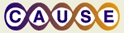# A ducks story- introducing the idea of testing (statistical) hypothesesThis material was originally developed through CAUSE
as part of its collaboration with the SERC Pedagogic Service.

## Summary

By means of a simple story and a worksheet with questions we guide the students from research question to arriving at a conclusion. The whole process is simply reasoning, no formulas. We use the reasoning already done by the student to introduce the standard vocabulary of testing statistical hypotheses (null & alternative hypotheses, p-value, type I and type II error, significance level). Students need to be familiar with binomial distribution tables.
After the ducks story is finished, the class is asked to come up with their own research question, collect the data, do the hypotheses testing and answer their own research question. The teaching material is intended to be flexible depending of the time available. Instructors can choose to do just the interactive lecture type, interactive lecture + activity, or even add the optional material.

## Learning Goals

From the interactive lecture part, they will learn the rationale of testing statistical hypotheses in general and be prepared to deal more comfortably with the material on testing hypotheses in standard books. The small sample case for testing hypotheses about population proportions, not covered in some introductory books, is included. With the activity part, not only the knowledge acquired through the interactive lecture will be reinforced, but also they will review other topics such: categorical variables, the idea of population proportion, identifying a population, surveys and experiments and data collection.

## Context for Use

This worksheet has two parts (in the same document) :
1. Interactive lecture to introduce the ideas and vocabulaty of hypotheses testing
2. Activity to apply what they learned in the interactive lecture <\li>
It can be used in an introductory statistics course as the first thing to do when we need to cover testing hypotheses. The interactive lecture can be covered in one class. The data for the activity can be collected after the class and the analysis can be done in the next class, but variations could be done. The way I apply the interactive lecture part is to allow time for individual reading, group discussion and discussion with the whole class after each section of the worksheet. At the end of that period the whole class can discuss and decide what new research question they want to work with, collect the data after class and discuss the analysis during the following class. It is appropriate for a intro stat class and no equipment (other than binomial tables either in paper or produced by software) is needed. The activity part is recommended but if time is short even doing just the interactive lecture part will be beneficial. Some instructors might want to cover the optional material also. There are two small documents with optional material: the extension to the large sample case and sample size and type I/II probability errors issues.

## Description and Teaching Materials

A worksheet with two parts: an interactive lecture and an activity. There are also two additional documents with optional topics.
Worksheet: Interactive Lecture and Activity (Microsoft Word 86kB May17 07)
Optional part 1: Large sample case (Microsoft Word 18kB May17 07)
Optional part 2: sample size and probability of error (Microsoft Word 33kB May17 07)

## Teaching Notes and Tips

The basic part of the interactive lecture (first 2 pages) give the basic ideas about writing hypotheses, p-values, arriving to a conclusion. The third page makes a revision of the thinking process and outlines the steps followed. It also introduces the concepts of type I and type II error and significance level. All the interactive lecture part can be covered in one class. Then comes the activity that is highly recommended to do, time permiting.<br > The two optional parts (in separate documents) can be used if time is available and depending on how far you want to go with the students in terms of hypotheses testing. I recommend to use group and class discussion combined with individual thinking and writing. This is the first thing I cover in my intro classes about hypotheses testing before I go to the text book material.
I have found also easy to adapt stories found in exercises in textbooks for the students to practice. You could change the ducks story for something more relevant to your own students.

## Assessment

The activity at the end can be used as assessment. Also any other story (extracted from the exercise section of your current text book) can be adapted to check if the following learning goals have been attained:
a) Is the student able to recognize the research question in a story and identify if that research question is about a population proportion?
b) Is the student able to translate the research question into null and alternative hypotheses?
c) Given the data, is the student able to calculate the p-value using the binomial table?
d) Is the student able to make the right decision about the null hypothesis?
e) Is the student able to answer the research question
f) Is the student able to identify the two types of error

## References and Resources

(for instructors) The reasons for the creation of this worksheet are stated in our paper Seier E. & Robe, C. (2002) Ducks and Green: An Introduction to the Ideas of Hypotheses Testing. Teaching Statistics. freely available from
http://www.blackwell-synergy.com/doi/pdf/10.1111/1467-9639.00094